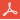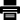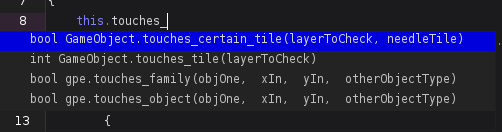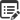# Tile Functions### 1.Quick Glance#

Tilesheet Functions are GPE based functions used to get data on a tilesheet and also used to find possible collisions of tiles within layers.Yes NoSuggest edit

### 2.Tile Based Functions#

#### int GPE.find_tile(int layerToCheck, int xPos, int yPos )

Returns the tilesheet id of the first tile the the coordinate touches. If none found, returns IS_NULL;

Scope: GPE

Return type :int

Parameters:

• int layerToCheck - Between 0 and 32 of the layer id to check on. Non-valid entries return IS_NULL.
• int xPos - the x-coordinate on the map(in pixels of where to search for the tile).
• int yPos - the x-coordinate on the map(in pixels of where to search for the tile).

#### int GPE.find_tile_in_box(int layerToCheck,  int x1,  int y1,  int x2, int y2 )

Returns the tilesheet id of the first tile the box touches. If none found, returns IS_NULL;

Scope: GPE

Return type :int

Parameters:

• int layerToCheck - Between 0 and 32 of the layer id to check on. Non-valid entries return IS_NULL.
• int x1- the first x-coordinate on the map(in pixels of where to search for the tile).
• int y1- the first y-coordinate on the map(in pixels of where to search for the tile).
• int x2- second the x-coordinate on the map(in pixels of where to search for the tile).
• int y2- second the y-coordinate on the map(in pixels of where to search for the tile).

#### bool GPE.find_matching_tile(int layerToCheck, int xPos,int yPos, int tileTypeToSearchFor )

Returns the true if a tile of the same tilesheet was detected at the inputted coordinates. If none found, returns false;

Scope: GPE

Return type :bool

Parameters:

• int layerToCheck - Between 0 and 32 of the layer id to check on. Non-valid entries return IS_NULL.
• int xPos - the x-coordinate on the map(in pixels of where to search for the tile).
• int yPos - the x-coordinate on the map(in pixels of where to search for the tile).
• int tileTypeToSearchFor - the tilesheet resource id to search for( value from 0 to X)

#### bool GPE.find_matching_tile_in_box(int layerToCheck,  int x1,  int y1,  int x2, int y2, int tileTypeToSearchFor )

Returns the true if a tile of the same tilesheet was detected in the inputted box. If none found, returns false;

Scope: GPE

Return type :bool

Parameters:

• int layerToCheck - Between 0 and 32 of the layer id to check on. Non-valid entries return IS_NULL.
• int x1- the first x-coordinate on the map(in pixels of where to search for the tile).
• int y1- the first y-coordinate on the map(in pixels of where to search for the tile).
• int x2- second the x-coordinate on the map(in pixels of where to search for the tile).
• int y2- second the y-coordinate on the map(in pixels of where to search for the tile).
• int tileTypeToSearchFor - the tilesheet resource id to search for( value from 0 to X)

#### int GameObject.touches_tile(int layerToCheck)

Returns the tilesheet id of the first tile the object touches. If none found, returns IS_NULL;

Scope: GameObject

Return type :int

Parameters:

• int layerToCheck - Between 0 and 32 of the layer id to check on. Non-valid entries return IS_NULL.

#### int GameObject.touches_certain_tile(int layerToCheck, needleTilesheet )

Returns true if the object touches the specific tilesheet on the given layer. Defaults to false for wrong parameters.

Scope: GameObject

Return type : int

Parameters:

• int layerToCheck - Between 0 and 32 of the layer id to check on. Non-valid entries return IS_NULL.
• int needleTilesheet - The tilesheet if to search for in the given layer.
Yes NoSuggest edit